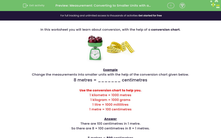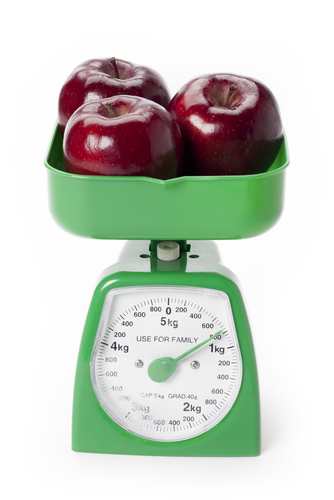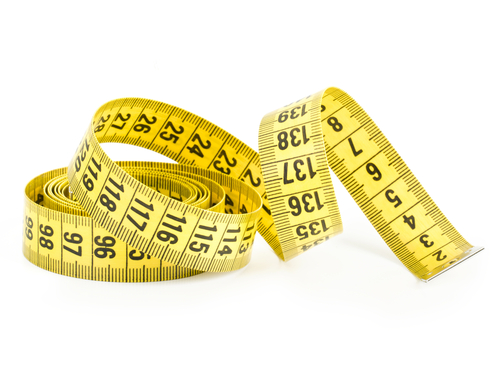# Convert Units of Length, Mass and Capacity

In this worksheet, students will convert units of length, mass and capacity.Key stage:  KS 2

Curriculum topic:   Measurement

Curriculum subtopic:   Measure, Compare, Add/Subtract Lengths, Mass, and Volume/Capacity

Difficulty level:#### Worksheet Overview

In this activity, we will learn about conversion, with the help of a conversion chart.1 kilometre = 1,000 metres

1 kilogram = 1,000 grams

1 litre = 1,000 millilitres

1 metre = 100 centimetres

Example

Convert 8 metres into centimetres.

We look at the conversion chart to see where it has metres and centimetres.

We can see that there are 100 centimetres in 1 metre.

So, in 8 metres there must be 8 x 100 = 800 cm

8 metres = 800 cm

Let's try another one.

Example

Convert 6,000 ml into litres.

Look at the chart to find where we have millilitres and litres.

We can see that there are 1,000 ml in a litre.

So, we can see that 6,000 ÷ 1,000 = 6 litres

6,000 ml = 6 litres

Let's try some questions now. If you want to look the introduction again, just click on the red help button on the screen.### What is EdPlace?

We're your National Curriculum aligned online education content provider helping each child succeed in English, maths and science from year 1 to GCSE. With an EdPlace account you’ll be able to track and measure progress, helping each child achieve their best. We build confidence and attainment by personalising each child’s learning at a level that suits them.

Get started••••